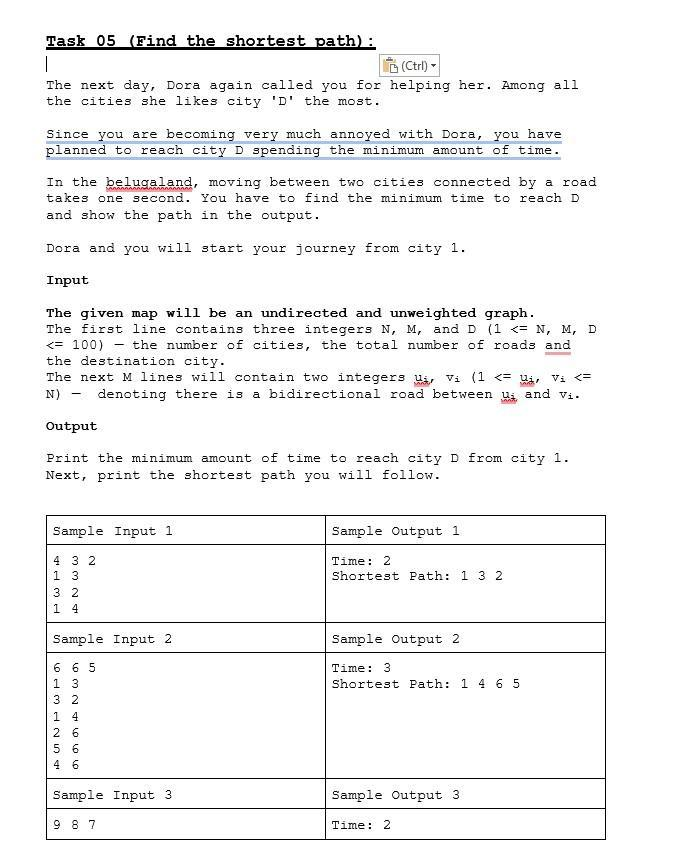# Question must use python   Task 05 (Find the shortest path): The next day, Dora again called you for helping her. Among all the cities she likes city 'D' the most. Since you are becoming very much annoyed with Dora, you have planned to reach city $$D$$ spending the minimum amount of time. In the belugaland, moving between two cities connected by a road takes one second. You have to find the minimum time to reach $$D$$ and show the path in the output. Dora and you will start your journey from city 1. Input The given map will be an undirected and unweighted graph. The first line contains three integers $$N, M$$, and $$D(1<=N, M, D$$ $$<=100$$ ) - the number of cities, the total number of roads and the destination city. The next $$M$$ lines will contain two integers $$u_{i}, v_{i}\left(1<=u_{i}, v_{i}<=\right.$$ N) - denoting there is a bidirectional road between $$u_{i}$$ and $$v_{i}$$. Output Print the minimum amount of time to reach city D from city 1. Next, print the shortest path you will follow.OAYKH7 The Asker · Computer Science

must use pythonTranscribed Image Text: Task 05 (Find the shortest path): The next day, Dora again called you for helping her. Among all the cities she likes city 'D' the most. Since you are becoming very much annoyed with Dora, you have planned to reach city $$D$$ spending the minimum amount of time. In the belugaland, moving between two cities connected by a road takes one second. You have to find the minimum time to reach $$D$$ and show the path in the output. Dora and you will start your journey from city 1. Input The given map will be an undirected and unweighted graph. The first line contains three integers $$N, M$$, and $$D(1<=N, M, D$$ $$<=100$$ ) - the number of cities, the total number of roads and the destination city. The next $$M$$ lines will contain two integers $$u_{i}, v_{i}\left(1<=u_{i}, v_{i}<=\right.$$ N) - denoting there is a bidirectional road between $$u_{i}$$ and $$v_{i}$$. Output Print the minimum amount of time to reach city D from city 1. Next, print the shortest path you will follow.
More
Transcribed Image Text: Task 05 (Find the shortest path): The next day, Dora again called you for helping her. Among all the cities she likes city 'D' the most. Since you are becoming very much annoyed with Dora, you have planned to reach city $$D$$ spending the minimum amount of time. In the belugaland, moving between two cities connected by a road takes one second. You have to find the minimum time to reach $$D$$ and show the path in the output. Dora and you will start your journey from city 1. Input The given map will be an undirected and unweighted graph. The first line contains three integers $$N, M$$, and $$D(1<=N, M, D$$ $$<=100$$ ) - the number of cities, the total number of roads and the destination city. The next $$M$$ lines will contain two integers $$u_{i}, v_{i}\left(1<=u_{i}, v_{i}<=\right.$$ N) - denoting there is a bidirectional road between $$u_{i}$$ and $$v_{i}$$. Output Print the minimum amount of time to reach city D from city 1. Next, print the shortest path you will follow.